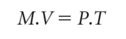# identity

(redirected from Identity (disambiguation))
Also found in: Dictionary, Thesaurus, Medical, Legal, Encyclopedia.

## identity

a means of portraying arithmetically the enduring equality between two (or more) VARIABLES that are equal by definition. For example, £1 = 100p (or \$1 = 100¢), and no matter how many pounds (or dollars) we have, they can always be converted into pennies (or cents) by multiplying by 100. Identities are generally given a three-bar ‘identity’ sign (=) to indicate that the value to the left of the three bars is identical to the value to the right of the sign. The QUANTITY THEORY OF MONEY is one of the best-known examples of an identity in economics, written as:where M is the money stock, V is the velocity of circulation of money, P is the general price level and T is the number of transactions undertaken. See EQUATION.

Collins Dictionary of Economics, 4th ed. © C. Pass, B. Lowes, L. Davies 2005
Site: Follow: Share:
Open / Close